# The Inspection Paradox is Everywhere¶

Allen Downey 2019

In :
import numpy as np
import pandas as pd
import matplotlib.pyplot as plt
import seaborn as sns

from empiricaldist import Pmf

from utils import decorate

In :
# set the random seed so we get the same results every time
np.random.seed(17)

In :
# make the directory for the figures
import os

if not os.path.exists('inspection'):
!mkdir inspection


## Class size¶

Here's the data summarizing the distribution of undergraduate class sizes at Purdue University in 2013-14.

In :
# Class size data originally from
# now available from

sizes = [(1, 1),
(2, 9),
(10, 19),
(20, 29),
(30, 39),
(40, 49),
(50, 99),
(100, 300)]

counts = [138, 635, 1788, 1979, 796, 354, 487, 333]


I generate a sample from this distribution, assuming a uniform distribution in each range and an upper bound of 300.

In :
def generate_sample(sizes, counts):
"""Generate a sample from a distribution.

sizes: sequence of (low, high) pairs
counts: sequence of integers

returns: NumPy array
"""
t = []
for (low, high), count in zip(sizes, counts):
print(count, low, high)
sample = np.random.randint(low, high+1, count)
t.extend(sample)
return np.array(t)


The "unbiased" sample is as seen by the college, with each class equally likely to be in the sample.

In :
unbiased = generate_sample(sizes, counts)

138 1 1
635 2 9
1788 10 19
1979 20 29
796 30 39
354 40 49
487 50 99
333 100 300


To generate a biased sample, we use the values themselves as weights and resample with replacement.

In :
def resample_weighted(sample, weights):
"""Resample values from sample with the given weights.

sample: NumPy array
weights: NumPy array

returns: NumPy array
"""
n = len(sample)
p = weights / np.sum(weights)
return np.random.choice(sample, n, p=p)

In :
biased = resample_weighted(unbiased, unbiased)


To plot the distribution, I use KDE to estimate the density function, then evaluate it over the given sequence of xs.

In :
from scipy.stats import gaussian_kde

def kdeplot(sample, xs, label=None, **options):
"""Use KDE to plot the density function.

sample: NumPy array
xs: NumPy array
label: string
"""
density = gaussian_kde(sample, **options).evaluate(xs)
plt.plot(xs, density, label=label)
decorate(ylabel='Relative likelihood')


The following plot shows the distribution of class size as seen by the Dean, and as seen by a sample of students.

In :
xs = np.arange(1, 300)
kdeplot(unbiased, xs, 'Reported by the Dean')
kdeplot(biased, xs, 'Reported by students')

decorate(xlabel='Class size',
title='Distribution of class sizes')

plt.savefig('inspection/class_size.png', dpi=150)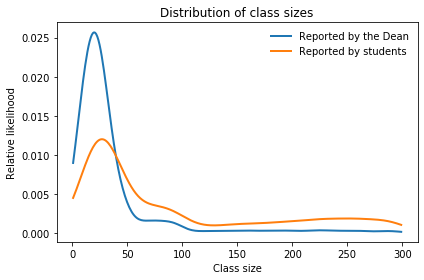Here are the means of the unbiased and biased distributions.

In :
np.mean(unbiased)

Out:
34.423041474654376
In :
np.mean(biased)

Out:
89.19170506912442
In :
from empiricaldist import Cdf

def cdfplot(sample, xs, label=None, **options):
"""Plot the CDF of the sample.

sample: NumPy array
xs: NumPy array (ignored)
label: string
"""
cdf = Cdf.from_seq(sample, **options)
cdf.plot(label=label)
decorate(ylabel='CDF')

In :
xs = np.arange(1, 300)
cdfplot(unbiased, xs, 'Reported by the Dean')
cdfplot(biased, xs, 'Reported by students')

decorate(xlabel='Class size',
title='Distribution of class sizes')

plt.savefig('inspection/class_size.png', dpi=150)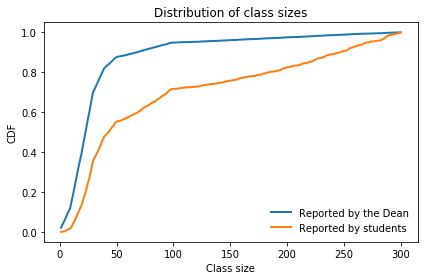## Red Line¶

Here are times between trains in seconds.

In :
unbiased = [
428.0, 705.0, 407.0, 465.0, 433.0, 425.0, 204.0, 506.0, 143.0, 351.0,
450.0, 598.0, 464.0, 749.0, 341.0, 586.0, 754.0, 256.0, 378.0, 435.0,
176.0, 405.0, 360.0, 519.0, 648.0, 374.0, 483.0, 537.0, 578.0, 534.0,
577.0, 619.0, 538.0, 331.0, 186.0, 629.0, 193.0, 360.0, 660.0, 484.0,
512.0, 315.0, 457.0, 404.0, 740.0, 388.0, 357.0, 485.0, 567.0, 160.0,
428.0, 387.0, 901.0, 187.0, 622.0, 616.0, 585.0, 474.0, 442.0, 499.0,
437.0, 620.0, 351.0, 286.0, 373.0, 232.0, 393.0, 745.0, 636.0, 758.0,
]


Here's the same data in minutes.

In :
unbiased = np.array(unbiased) / 60


We can use the same function to generate a biased sample.

In :
biased = resample_weighted(unbiased, unbiased)


And plot the results.

In :
xs = np.linspace(1, 16.5, 101)
kdeplot(unbiased, xs, 'Seen by MBTA')
kdeplot(biased, xs, 'Seen by passengers')

decorate(xlabel='Time between trains (min)',
title='Distribution of time between trains')

plt.savefig('inspection/red_line.png', dpi=150)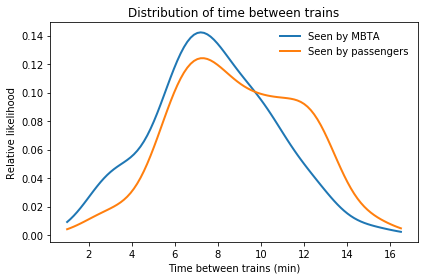In :
xs = np.linspace(1, 16.5, 101)
cdfplot(unbiased, xs, 'Seen by MBTA')
cdfplot(biased, xs, 'Seen by passengers')

decorate(xlabel='Time between trains (min)',
title='Distribution of time between trains')

plt.savefig('inspection/red_line.png', dpi=150)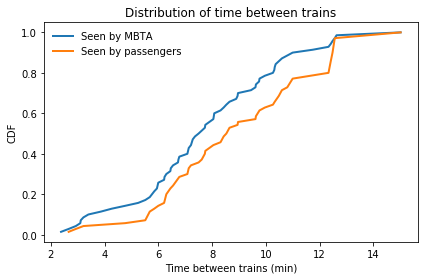Here are the means of the distributions and the percentage difference.

In :
np.mean(biased), np.mean(unbiased)

Out:
(9.207857142857144, 7.7680952380952375)
In :
(np.mean(biased) - np.mean(unbiased)) /  np.mean(unbiased) * 100

Out:
18.53429779930119

## Social network¶

In :
import networkx as nx

"""Read a graph from a file.

filename: string

return: nx.Graph
"""
G = nx.Graph()
return G

In :
# https://snap.stanford.edu/data/facebook_combined.txt.gz

n = len(fb)
m = len(fb.edges())
n, m

Out:
(4039, 88234)

The unbiased sample is the number of friends for each user.

In :
unbiased = [fb.degree(node) for node in fb]
len(unbiased)

Out:
4039
In :
np.max(unbiased)

Out:
1045

We can use the same function to generate a biased sample.

In :
biased = resample_weighted(unbiased, unbiased)


And generate the plot.

In :
xs = np.linspace(0, 300, 101)
kdeplot(unbiased, xs, 'Random sample of people')
kdeplot(biased, xs, 'Random sample of friends')

decorate(xlabel='Number of friends in social network',
title='Distribution of social network size')

plt.savefig('inspection/social.png', dpi=150)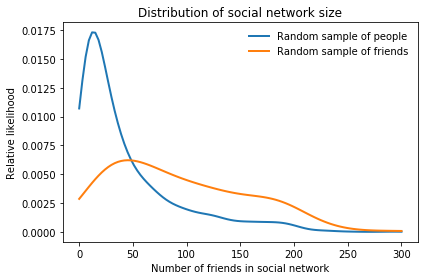In :
xs = np.linspace(0, 300, 101)
cdfplot(unbiased, xs, 'Random sample of people')
cdfplot(biased, xs, 'Random sample of friends')

decorate(xlabel='Number of friends in social network',
title='Distribution of social network size',
xlim=[-10, 310])

plt.savefig('inspection/social.png', dpi=150)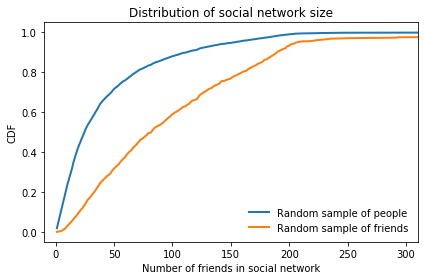Here are the means of the distributions.

In :
np.mean(biased), np.mean(unbiased)

Out:
(108.5679623669225, 43.69101262688784)

And the probability that the friend of a user has more friends than the user.

In :
np.mean(biased > unbiased)

Out:
0.7655360237682595

## Relay race¶

The following function read the data from the 2010 James Joyce Ramble 10K, where I ran my personal record time.

In :
import relay
unbiased = relay.GetSpeeds(results)


In this case, the weights are related to the difference between each element of the sample and the hypothetical speed of the observer.

In :
weights = np.abs(np.array(unbiased) - 7)
biased = resample_weighted(unbiased, weights)


And here's the plot.

In :
xs = np.linspace(3, 11, 101)
kdeplot(unbiased, xs, 'Seen by spectator')
kdeplot(biased, xs, 'Seen by runner at 7 mph', bw_method=0.2)

decorate(xlabel='Running speed (mph)',
title='Distribution of running speed')

plt.savefig('inspection/relay.png', dpi=150)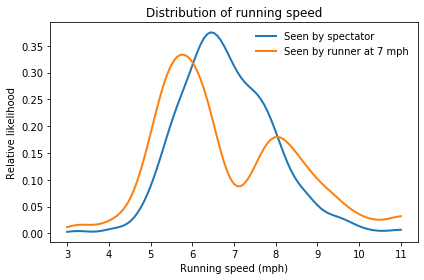In :
xs = np.linspace(3, 11, 101)
cdfplot(unbiased, xs, 'Seen by spectator')
cdfplot(biased, xs, 'Seen by runner at 7 mph')

decorate(xlabel='Running speed (mph)',
title='Distribution of running speed')

plt.savefig('inspection/relay.png', dpi=150)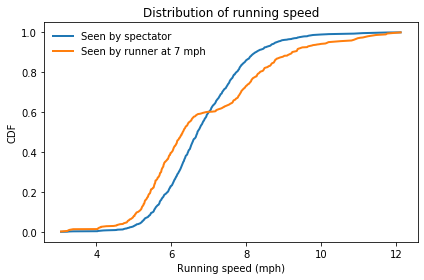## Prison sentences¶

First we read the data from the Bureau of Prisons web page.

In :
tables = pd.read_html('BOP Statistics_ Sentences Imposed.html')
df = tables
df

Out:
Sentence # of Inmates % of Inmates
0 0 to 1 year* 5155 2.3 %
1 > 1 year to < 3 years** 18619 11.3%
2 3 years to < 5 years 17897 10.9%
3 5 years to < 10 years 41887 25.4%
4 10 years to < 15 years 34995 21.3%
5 15 years to < 20 years 18674 11.3%
6 20 years or more but < Life 22738 13.8%
7 Life 4600 2.8%

Here are the low and I sentences for each range. I assume that the minimum sentence is about a week, that sentences "less than life" are 40 years, and that a life sentence is between 40 and 60 years.

In :
sentences = [(0.02, 1),
(1, 3),
(3, 5),
(5, 10),
(10, 15),
(15, 20),
(20, 40),
(40, 60)]


We can get the counts from the table.

In :
counts = df['# of Inmates']


Here's a different version of generate_sample for a continuous quantity.

In :
def generate_sample(sizes, counts):
"""Generate a sample from a distribution.

sizes: sequence of (low, high) pairs
counts: sequence of integers

returns: NumPy array
"""
t = []
for (low, high), count in zip(sizes, counts):
print(count, low, high)
sample = np.random.uniform(low, high, count)
t.extend(sample)
return np.array(t)


In this case, the data are biased.

In :
biased = generate_sample(sentences, counts)

5155 0.02 1
18619 1 3
17897 3 5
41887 5 10
34995 10 15
18674 15 20
22738 20 40
4600 40 60


So we have to unbias them with weights inversely proportional to the values.

Prisoners in federal prison typically serve 85% of their nominal sentence. We can take that into account in the weights.

In :
weights = 1 / (0.85 * np.array(biased))


Here's the unbiased sample.

In :
unbiased = resample_weighted(biased, weights)


And the plotted distributions.

In :
xs = np.linspace(0, 60, 101)
kdeplot(unbiased, xs, 'Seen by judge', bw_method=0.5)
kdeplot(biased, xs, 'Seen by prison visitor', bw_method=0.5)

decorate(xlabel='Prison sentence (years)',
title='Distribution of federal prison sentences')

plt.savefig('inspection/orange.png', dpi=150)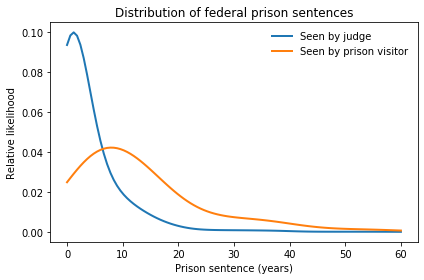In :
xs = np.linspace(0, 60, 101)
cdfplot(unbiased, xs, 'Seen by judge')
cdfplot(biased, xs, 'Seen by prison visitor')

decorate(xlabel='Prison sentence (years)',
title='Distribution of federal prison sentences')

plt.savefig('inspection/orange.png', dpi=150)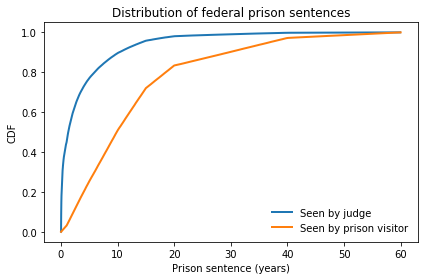We can also compute the distribution of sentences as seen by someone at the prison for 13 months.

In :
x = 0.85 * unbiased
y = 13 / 12

weights = x + y


Here's the sample.

In :
kerman = resample_weighted(unbiased, weights)


And here's what it looks like.

In :
xs = np.linspace(0, 60, 101)
kdeplot(unbiased, xs, 'Seen by judge', bw_method=0.5)
kdeplot(kerman, xs, 'Seen by Kerman', bw_method=0.5)
kdeplot(biased, xs, 'Seen by visitor', bw_method=0.5)

decorate(xlabel='Prison sentence (years)',
title='Distribution of federal prison sentences')

plt.savefig('inspection/orange.png', dpi=150)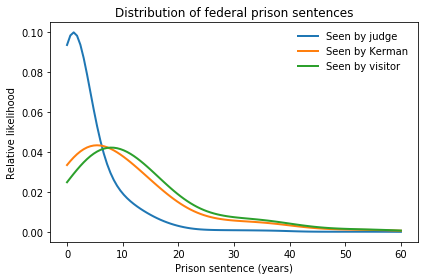In :
xs = np.linspace(0, 60, 101)
cdfplot(unbiased, xs, 'Seen by judge')
cdfplot(kerman, xs, 'Seen by Kerman')
cdfplot(biased, xs, 'Seen by visitor')

decorate(xlabel='Prison sentence (years)',
title='Distribution of federal prison sentences')

plt.savefig('inspection/orange.png', dpi=150)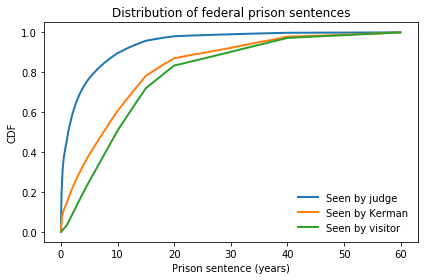In the unbiased distribution, almost half of prisoners serve less than one year.

In :
np.mean(unbiased<1)

Out:
0.44996809771215024

But if we sample the prison population, barely 3% are short timers.

In :
np.mean(biased<1)

Out:
0.0313250083553611

Here are the means of the distributions.

In :
np.mean(unbiased)

Out:
3.5762182609236373
In :
np.mean(biased)

Out:
12.778002004195422
In :
np.mean(kerman)

Out:
10.455078798407829

## The dartboard problem¶

In [ ]:
from matplotlib.patches import Circle

def draw_dartboard():
ax = plt.gca()

c1 = Circle((0, 0), 170, color='C3', alpha=0.3)
c2 = Circle((0, 0), 160, color='white')
c3 = Circle((0, 0), 107, color='C3', alpha=0.3)
c4 = Circle((0, 0), 97, color='white')
c5 = Circle((0, 0), 16, color='C3', alpha=0.3)
c6 = Circle((0, 0), 6, color='white')
for circle in [c1, c2, c3, c4, c5, c6]:
plt.axis('equal')


In :
draw_dartboard()
plt.text(0, 10, '25 ring')
plt.text(0, 110, 'triple ring')
plt.text(0, 170, 'double ring')

plt.savefig('inspection/darts0.png', dpi=150)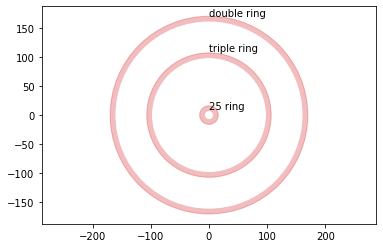In :
sigma = 50
n = 100
error_x = np.random.normal(0, sigma, size=(n))
error_y = np.random.normal(0, sigma, size=(n))

In :
draw_dartboard()
plt.plot(error_x, error_y, '.')
plt.savefig('inspection/darts1.png', dpi=150)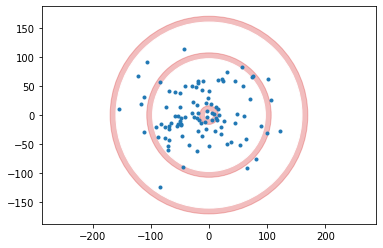In :
sigma = 50
n = 10000
error_x = np.random.normal(0, sigma, size=(n))
error_y = np.random.normal(0, sigma, size=(n))

In :
import numpy as np
import seaborn as sns
import matplotlib.pyplot as pl

ax = sns.kdeplot(error_x, error_y, shade=True, cmap="PuBu")
ax.collections.set_alpha(0)
plt.axis([-240, 240, -175, 175])

decorate(xlabel='x distance from center (mm)',
ylabel='y distance from center (mm)',
title='Estimated density')

plt.savefig('inspection/darts2.png', dpi=150)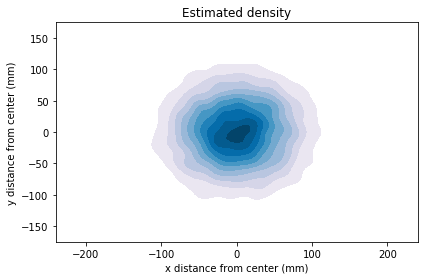In :
rs = np.hypot(error_x, error_y)

In :
np.random.seed(18)
sigma = 50
n = 10000
error_x = np.random.normal(0, sigma, size=(n))
error_y = np.random.normal(0, sigma, size=(n))

In :
xs = np.linspace(-200, 200, 101)

#ys = np.exp(-(xs/sigma)**2/2)
#pmf = Pmf(ys, index=xs)
#pmf.normalize()
#pmf.plot(color='gray')

unbiased = error_x
biased = resample_weighted(unbiased, np.abs(unbiased))

kdeplot(unbiased, xs, 'Density at a point')
kdeplot(biased, xs, 'Total density in a ring')
#kdeplot(rs, xs, 'Total density in a ring')

decorate(xlabel='Distance from center (mm)',
ylabel='Density',
xlim=[0, 210])

plt.savefig('inspection/darts3.png', dpi=150)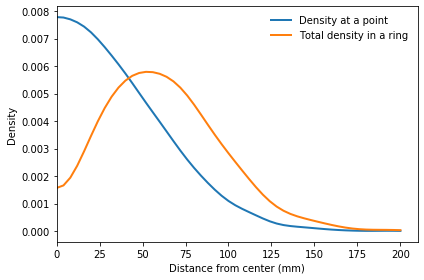In :
xs = np.linspace(0, 200, 101)
unbiased = np.abs(error_x)
biased = resample_weighted(unbiased, unbiased)

cdfplot(unbiased, xs, 'Density at a point')
cdfplot(biased, xs, 'Total density in a ring')

decorate(xlabel='Distance from center (mm)',
ylabel='Density')

plt.savefig('inspection/darts4.png', dpi=150)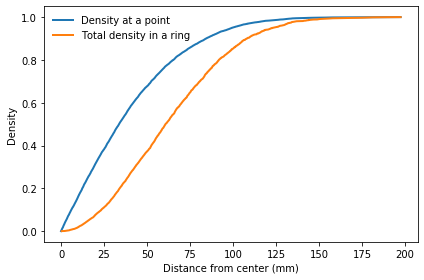In :
triple = (biased > 97) & (biased < 107)
triple.mean() * 100

Out:
6.15
In :
ring50 = (biased > 6) & (biased < 16)
ring50.mean() * 100

Out:
4.6
In :
double = (biased > 160) & (biased < 170)
double.mean() * 100

Out:
0.22
In :
bull = (biased < 6)
bull.mean() * 100

Out:
0.76
In [ ]: